Week 3 Combinational Logic Blocks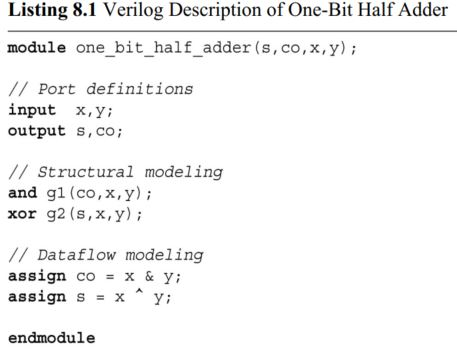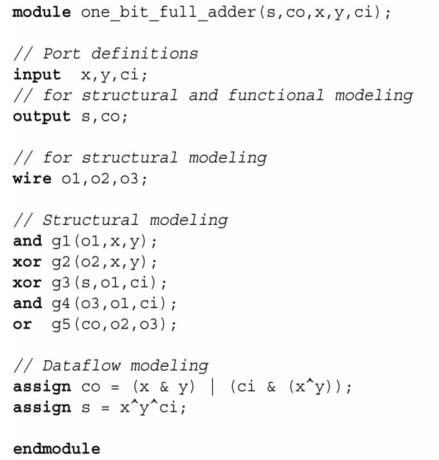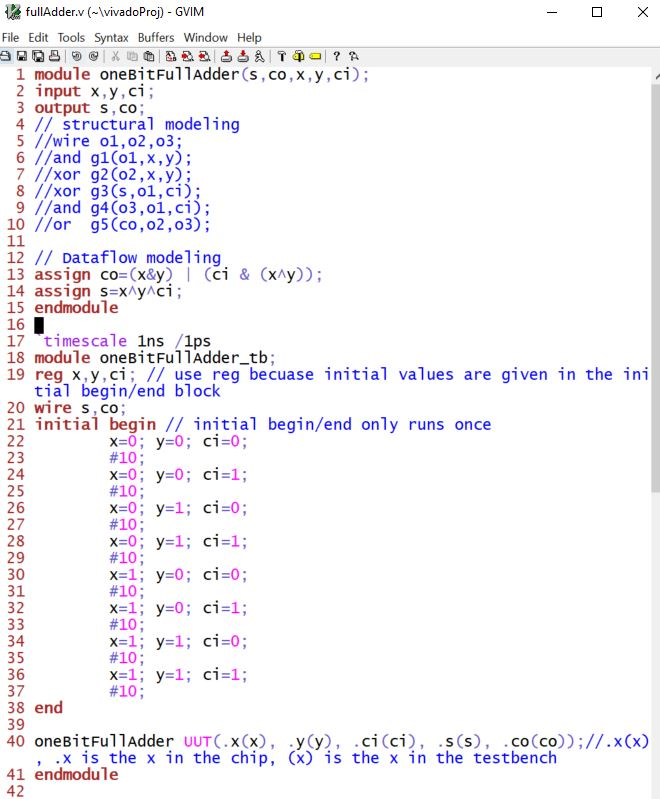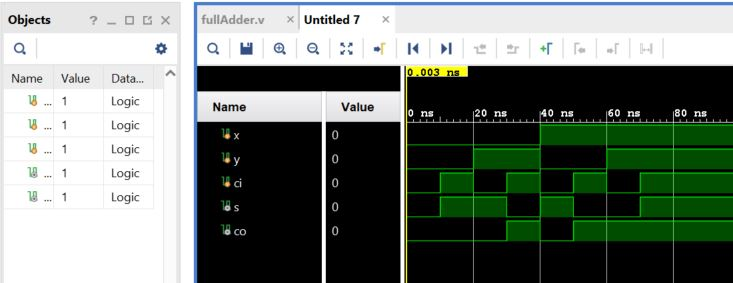In the full adder testbench, when there are many simulation entries, you can use a for loop to simplify it.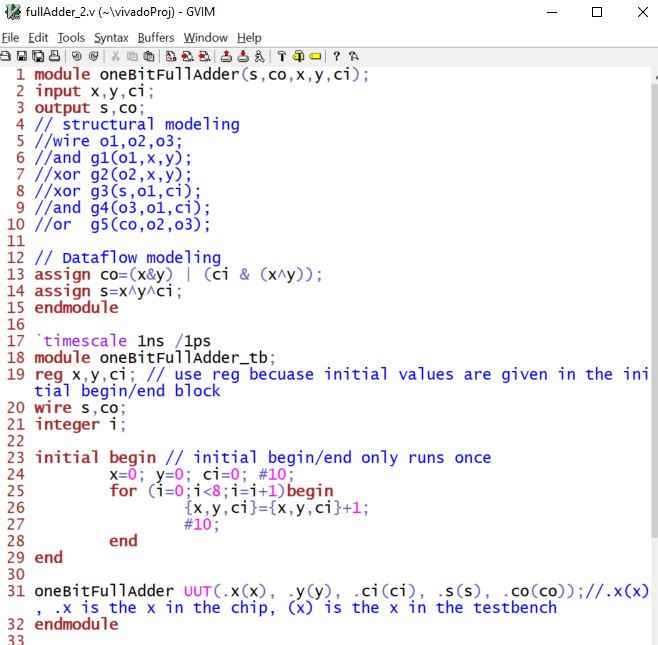2. Comparators

We may need to compare the magnitude of two binary numbers to obtain their status. Here, the first number may be greater than the second. The two numbers may be equal. Or, the first number may be less than the second. To achieve this goal, we will need a comparator. We can explain the comparison operation on two binary variables x and y (each being one bit) using the truth table presented in the following truth table. Here, g, e, and l stand for greater, equal, and less, respectively.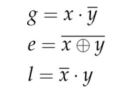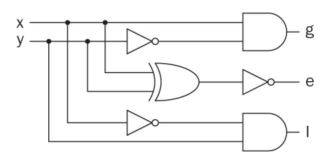In Verilog: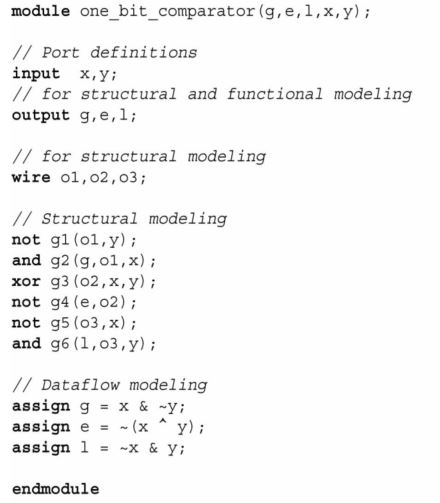3. Conditional statement in Verilog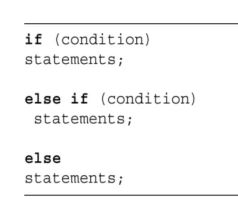A 4-bit comparator

An N-bit comparator can be constructed by the 'if' keyword. Here, two vectors each with four-bits (x and y) are compared and the result is written to another vector comp. If the first vector is greater than the second one, then comp=1. If the second vector is greater than the first one, then comp=1. Finally, if the two vectors are equal, then comp=1.Another example of the IF Statement (not a comparator):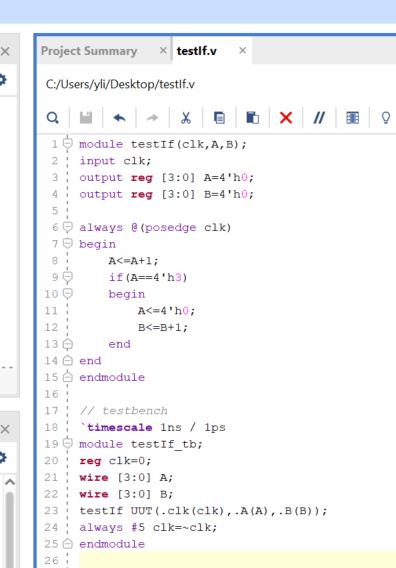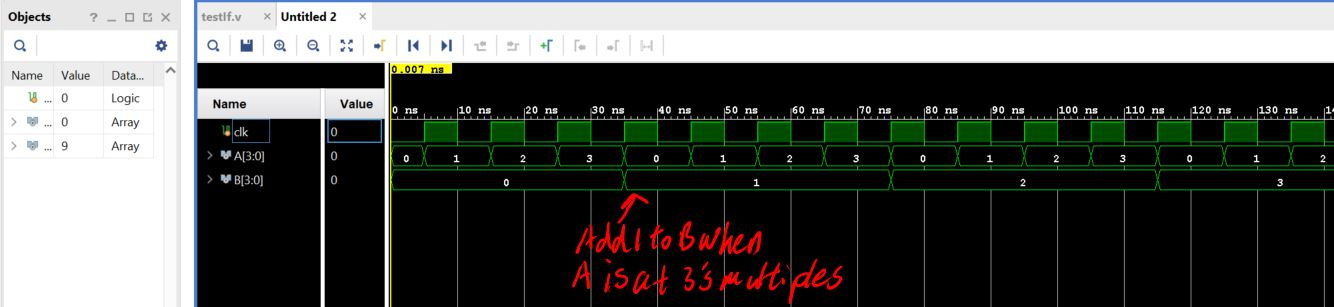If I remove the 'begin/end' statement in the IF statement and keep 'B<=B+1' below the 'A<=4'h0' line, B will have an increment of 1 at each cycle.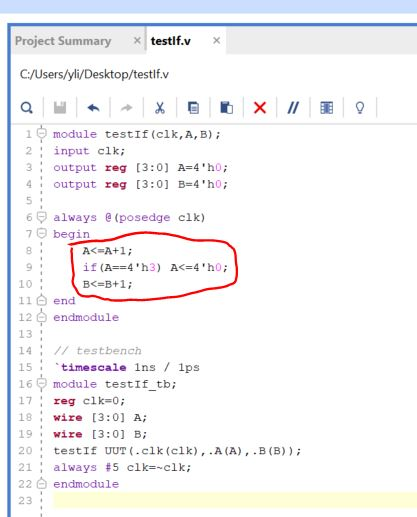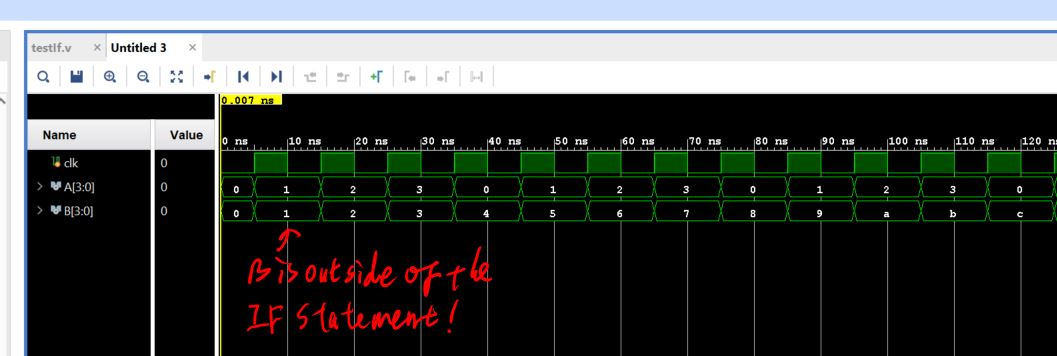This example tells you that the 'begin/end' block defines the boundary of the IF statement if you have multiple lines there.

4. Decoders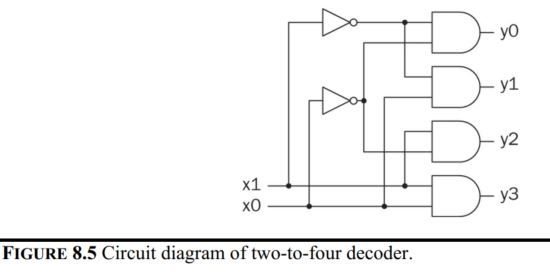In Verilog,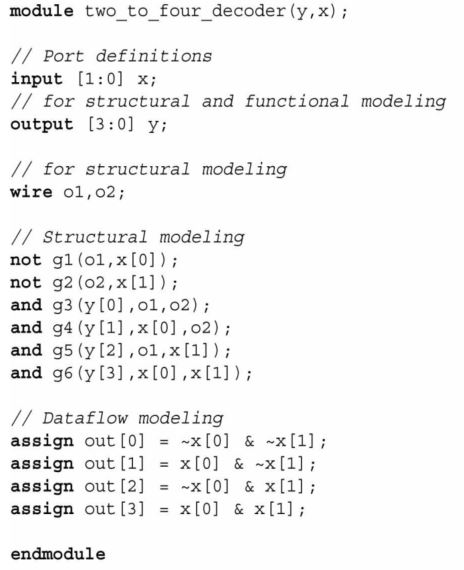The 'case' statement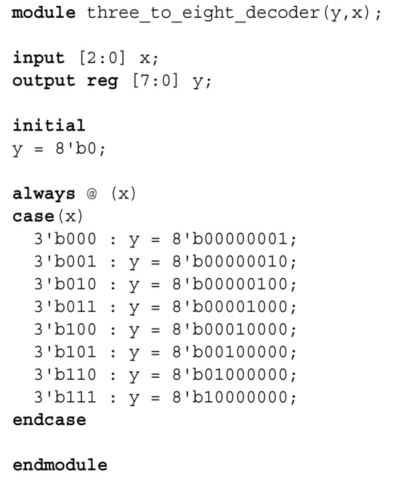5. Encoders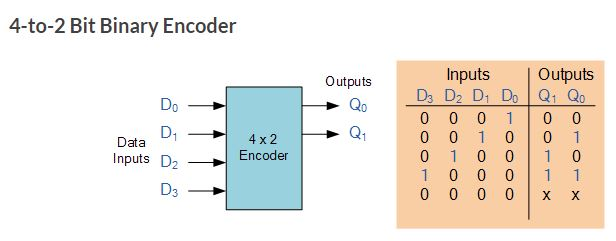One of the main disadvantages of standard digital encoders is that they can generate the wrong output code when there is more than one input present at logic level “1”. For example, if we make inputs D1 and D2 HIGH at logic “1” both at the same time, the resulting output is neither at “01” or at “10” but will be at “11” which is an output binary number that is different to the actual input present. Also, an output code of all logic “0”s can be generated when all of its inputs are at “0” OR when input D0 is equal to one. One simple way to overcome this problem is to “Prioritise” the level of each input pin. So if there is more than one input at logic level “1” at the same time, the actual output code would only correspond to the input with the highest designated priority. Then this type of digital encoder is known commonly as a Priority Encoder or P-encoder for short.

Priority encoders

The Priority Encoder solves the problems mentioned above by allocating a priority level to each input. The priority encoders output corresponds to the currently active input which has the highest priority. So when an input with a higher priority is present, all other inputs with a lower priority will be ignored. The priority encoder comes in many different forms with an example of an 8-input priority encoder along with its truth table shown below.Priority encoders output the highest order input first for example, if input lines “D2“, “D3” and “D5” are applied simultaneously the output code would be for input “D5” (“101”) as this has the highest order out of the 3 inputs. Once input “D5” had been removed the next highest output code would be for input “D3” (“011”), and so on. The truth table for a 8-to-3 bit priority encoder is given as: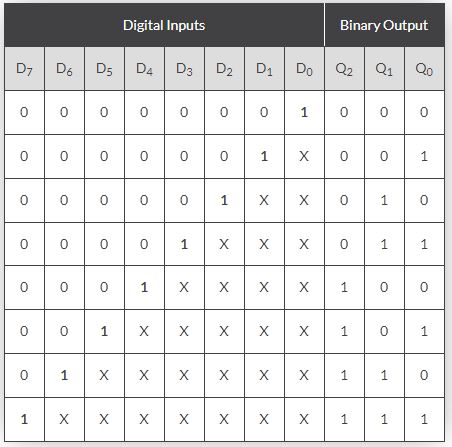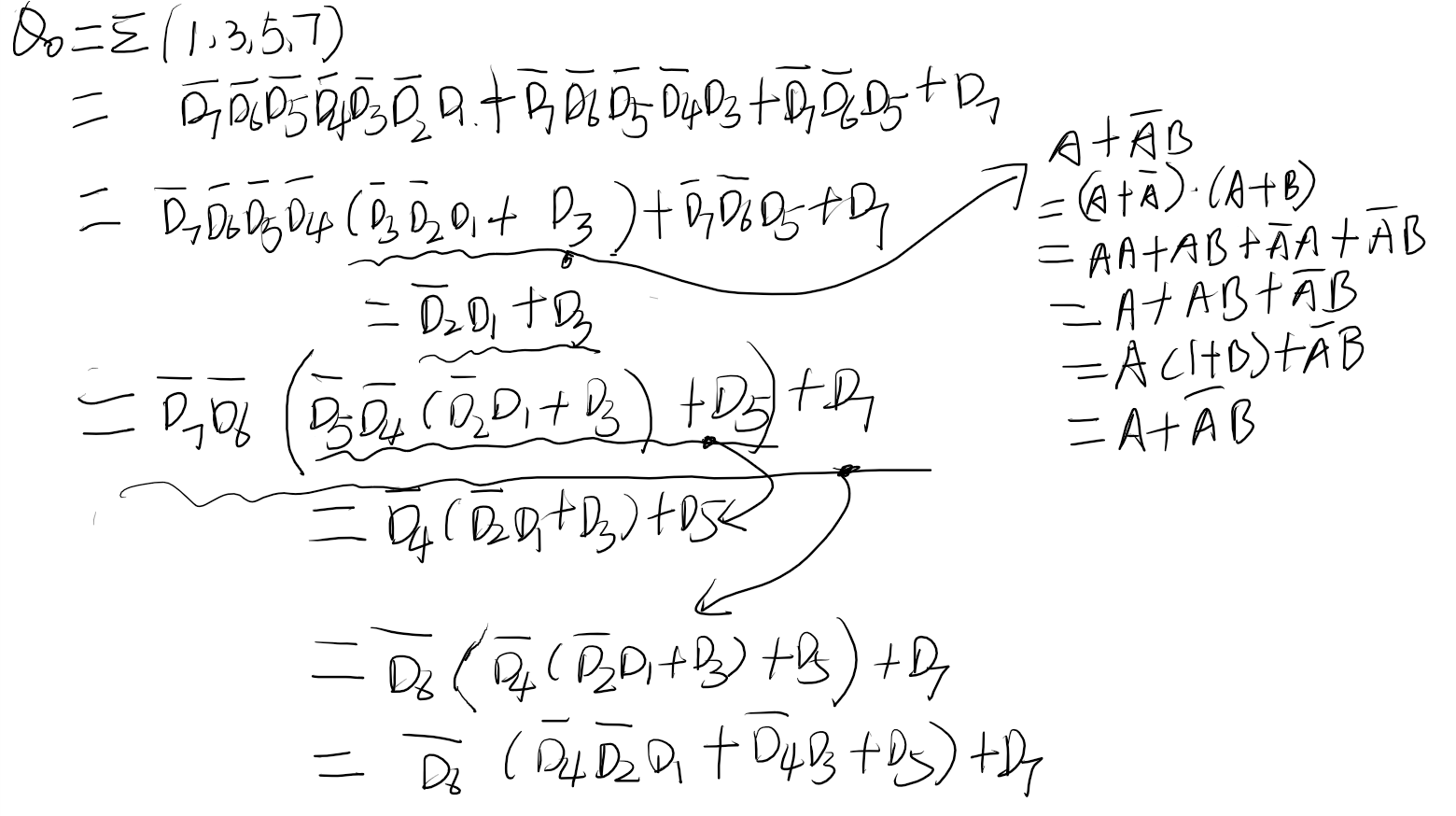What are Q1 and Q2?

Digital Encoder Applications Keyboard Encoder Priority encoders can be used to reduce the number of wires needed in a particular circuits or application that have multiple inputs. For example, assume that a microcomputer needs to read the 104 keys of a standard QWERTY keyboard where only one key would be pressed either “HIGH” or “LOW” at any one time.

One way would be to connect all 104 wires from the individual keys on the keyboard directly to the computers input but this would be impractical for a small home PC. Another alternative and better way would be to interface the keyboard to the PC using a priority encoder.

The 104 individual buttons or keys could be encoded into a standard ASCII code of only 7-bits (0 to 127 decimal) to represent each key or character of the keyboard and then input as a much smaller 7-bit B.C.D code directly to the computer. Keypad encoders such as the 74C923 20-key encoder are available to do just that.

Verilog implementation using the casex statement:Positional Encoders

Another more common application is in magnetic positional control as used on ships navigation or for robotic arm positioning etc. Here for example, the angular or rotary position of a compass is converted into a digital code by a 74LS148 8-to-3 line priority encoder and input to the systems computer to provide navigational data and an example of a simple 8 position to 3-bit output compass encoder is shown below. Magnets and reed switches could be used at each compass point to indicate the needles angular position.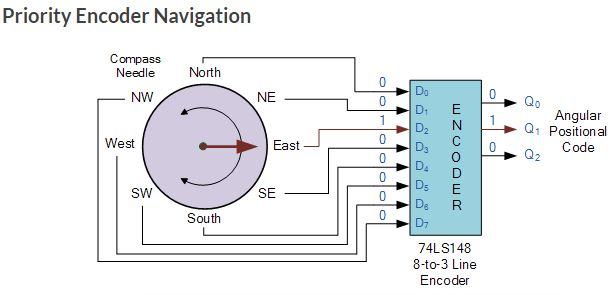6. Multiplexers

An eight-to-one multiplexerIn Verilog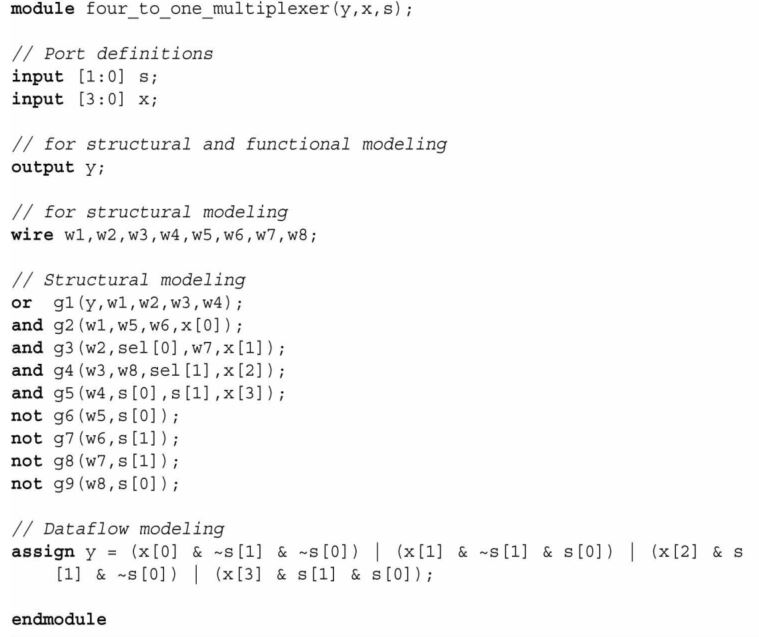The 'case' version.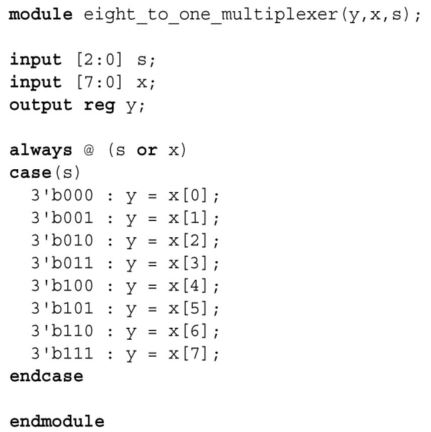7. Parity generators and checkers

While transferring or storing binary data, some bit values may change because of a physical effect or an unpredicted disturbance. To check whether such an undesired change has occurred or not, extra bits can be added to the data. This is called parity generation. The idea here is setting standard characteristics to data such that when a change occurs, it can be detected easily.

Even Parity Generator - The total number of 1's in input and the parity bit are even
Odd Parity Generator - The total number of 1's in input and the parity bit are odd

Even Parity Generator (make the total number of 1's even)
Let us assume that a 3-bit message is to be transmitted with an even parity bit. Let the three inputs A, B and C are applied to the circuit and output bit is the parity bit P. The total number of 1s must be even, to generate the even parity bit P. The figure below shows the truth table of even parity generator in which 1 is placed as parity bit in order to make all 1s as even when the number of 1s in the truth table is odd.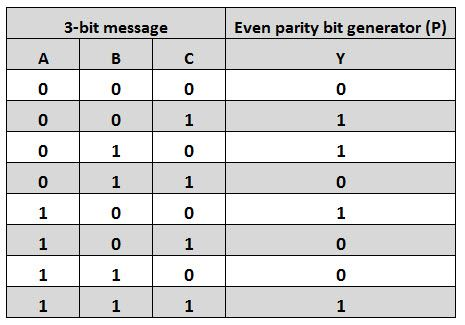Use a K Map to find the logic expression of the truth table:The above expression can be implemented by using two XOR gates. The logic diagram of even parity generator with two XOR gates is shown below. The three bit message along with the parity generated by this circuit which is transmitted to the receiving end where parity checker circuit checks whether any error is present or not.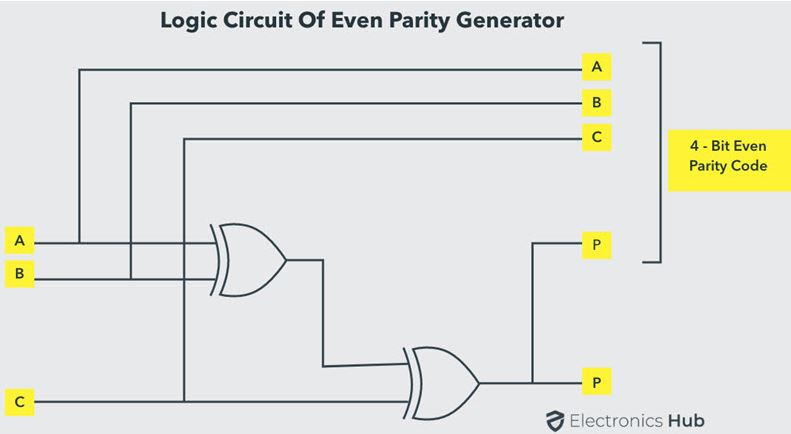In Verilog: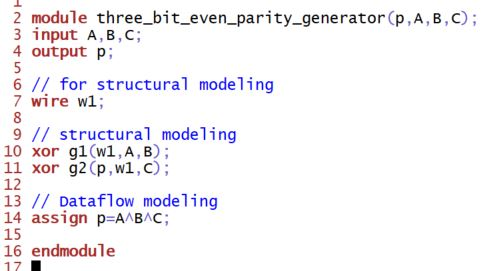Even Parity Checker

It is a logic circuit that checks for possible errors in the transmission. This circuit can be an even parity checker or odd parity checker depending on the type of parity generated at the transmission end. When this circuit is used as even parity checker, the number of input bits must always be even.

Even Parity Checker Consider that three input message along with even parity bit is generated at the transmitting end. These 4 bits are applied as input to the parity checker circuit, which checks the possibility of error on the data. Since the data is transmitted with even parity, four bits received at circuit must have an even number of 1s. If any error occurs, the received message consists of odd number of 1s. The output of the parity checker is denoted by PEC (Parity Error Check). The below table shows the truth table for the Even Parity Checker in which PEC = 1 if the error occurs, i.e., the four bits received have odd number of 1s and PEC = 0 if no error occurs, i.e., if the 4-bit message has even number of 1s.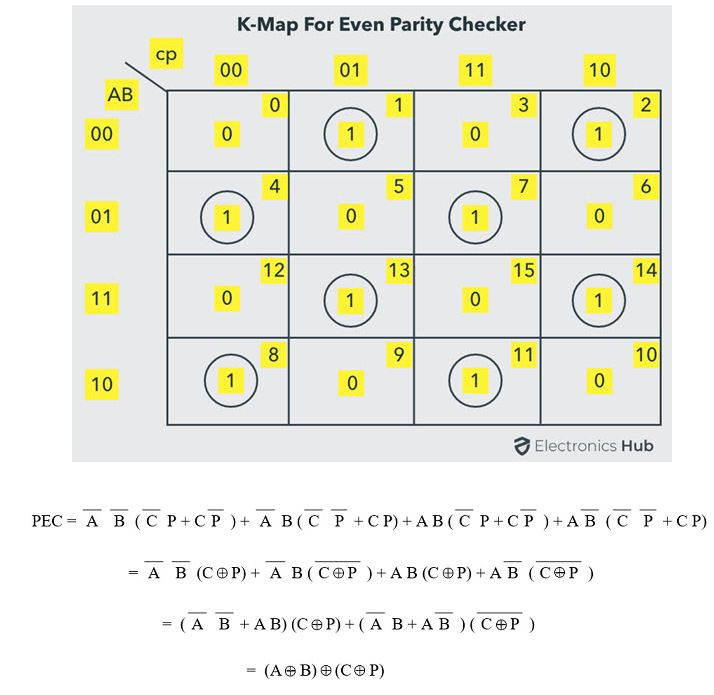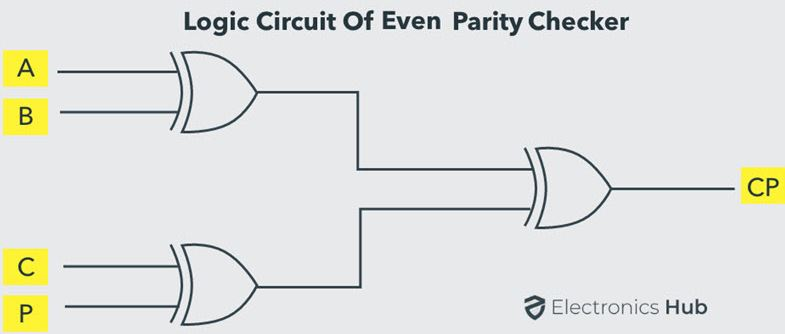Odd Parity Generator (make the total number of 1's odd)

Truth table: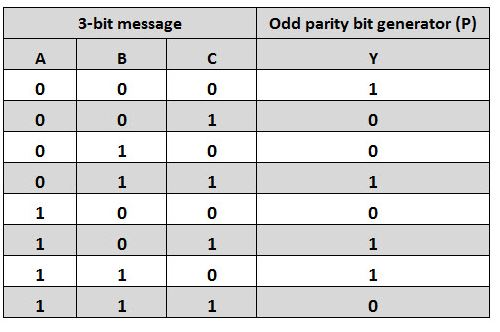Logic expression:Circuit:Odd Parity Checker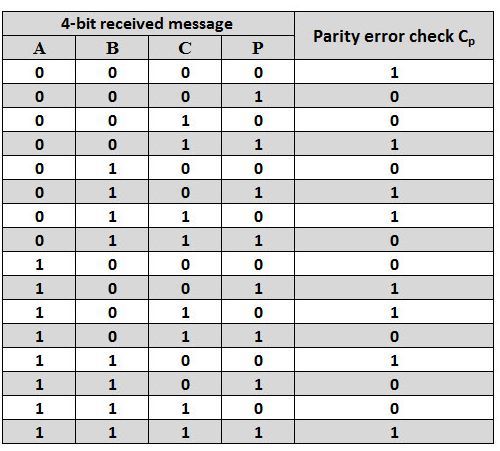Logic expression: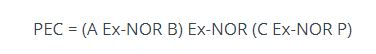The circuit:8. Improving the home alarm system

We can improve the home alarm system using a seven-segment display. When the system is active, the display will show character A. When it is closed, the display will show character O.

To do so, we should add a seven-segment display decoder module to the system. This module converts the provided hexadecimal number to the corresponding seven-segment display pattern.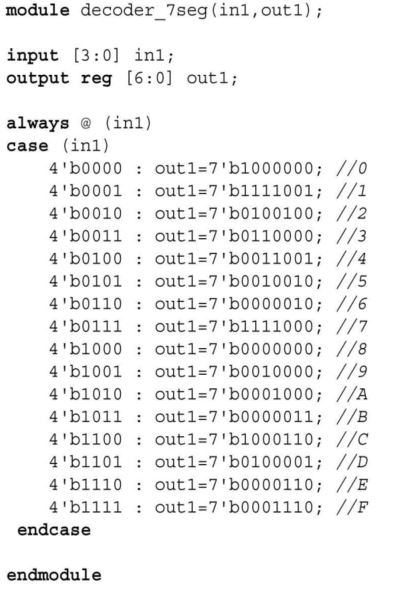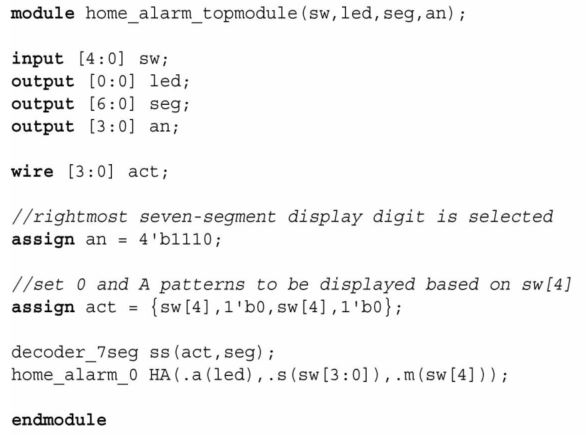Please refer to the last tutorial to implement the improved home alarm system on your Basys3 Board.

9. Improved car parking spot counting system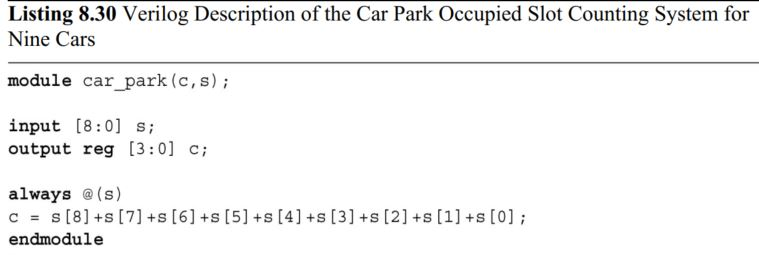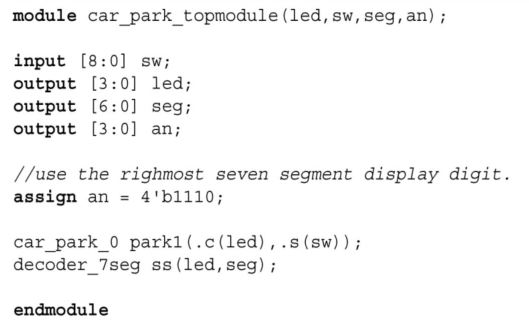--------------------